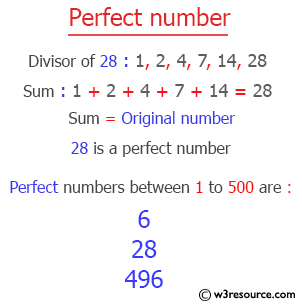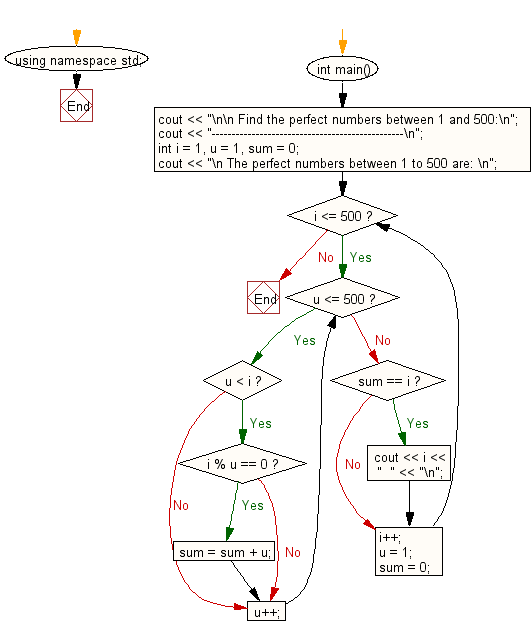﻿ C++ Exercises: Find the perfect numbers between 1 and 500 - w3resource# C++ Exercises: Find the perfect numbers between 1 and 500

## C++ For Loop: Exercise-4 with Solution

Write a program in C++ to find the perfect numbers between 1 and 500.

Pictorial Presentation:Sample Solution :-

C++ Code :

``````#include <iostream>
using namespace std;
int main()
{
cout << "\n\n Find the perfect numbers between 1 and 500:\n";
cout << "------------------------------------------------\n";
int i = 1, u = 1, sum = 0;
cout << "\n The perfect numbers between 1 to 500 are: \n";
while (i <= 500)
{
while (u <= 500)
{
if (u < i)
{
if (i % u == 0)
sum = sum + u;
}
u++;
}
if (sum == i) {
cout << i << "  " << "\n";
}
i++;
u = 1;
sum = 0;
}
}
``````

Sample Output:

``` Find the perfect numbers between 1 and 500:
------------------------------------------------

The perfect numbers between 1 to 500 are:
6
28
496
```

Flowchart:C++ Code Editor:

Contribute your code and comments through Disqus.

What is the difficulty level of this exercise?

﻿

## C++ Programming: Tips of the Day

What is a smart pointer and when should I use one?

This answer is rather old, and so describes what was 'good' at the time, which was smart pointers provided by the Boost library. Since C++11, the standard library has provided sufficient smart pointers types, and so you should favour the use of std::unique_ptr, std::shared_ptr and std::weak_ptr.

There was also std::auto_ptr. It was very much like a scoped pointer, except that it also had the "special" dangerous ability to be copied - which also unexpectedly transfers ownership.

It was deprecated in C++11 and removed in C++17, so you shouldn't use it.

```std::auto_ptr<MyObject> p1 (new MyObject());
std::auto_ptr<MyObject> p2 = p1; // Copy and transfer ownership.
// p1 gets set to empty!
p2->DoSomething(); // Works.
p1->DoSomething(); // Oh oh. Hopefully raises some NULL pointer exception.
```

Ref : https://bit.ly/3mc9GHE